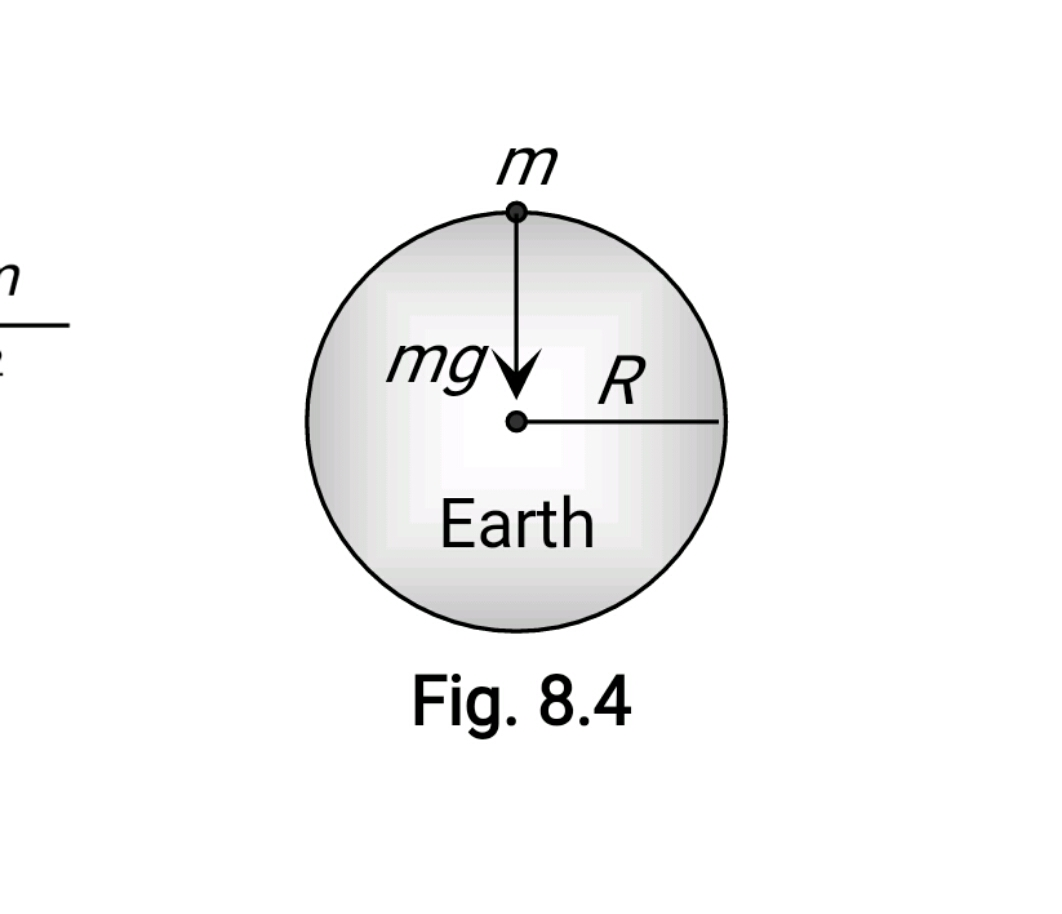#### Two planets of radii r1 and r2 are made from the same material. The ratio of the acceleration due to gravity g1/g2 at the surface of the two planets is Option 1)Option 2)Option 3)Option 4)As we learnt in

Acceleration due to gravity (g) -

Force extended by earth on a body is gravity.

Formula:gravitydensity of earthRadius of earth

- wherein

It's average value ison the surface of earthSince both are made of same material hence their densities are equalOption 1)This is correct option

Option 2)This is incorrect option

Option 3)This is incorrect option

Option 4)This is incorrect option